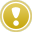English

MQL4 BookOperatorsAssignment Operator

# Assignment Operator

The assignment operator is the simplest and the most commonly used operator.

## Format of the Assignment Operator

Assignment operator represents a record that contains character "=" (equality sign). To the left of this equality sign we specify the name of a variable, to the right of it we give an expression. The assignment operator is ended with ";" (semicolon).

`  Variable = Expression;                          // Assignment operator`

You can distinguish the assignment operator from other lines in the text of the program by the presence of the equality sign. You can specify as an expression: a constant, a variable, a function call, or an expression as such.

## Execution of the Assignment OperatorCalculate the value of the expression to the right from the equality sign and assign the obtained value to the variable specified to the left of the equality sign.

The assignment operator, like any other operator, is executable. This means that the record composing the assignment operator is executed according to the rule. When executing the operator, the value of the right part is calculated and then assigned to the variable to the left of the equality sign. As a result of execution of the assignment operator, the variable in the left part always takes a new value; this value can be other than or the same as the preceding value of the variable. The expression in the right part of the assignment operator is calculated according to the order of operations (see Operations and Expressions).

## Examples of Assignment Operators

In an assignment operator, it is allowed to declare the type of a variable to the left of the equality sign:

`   int In = 3;                // The constant value is assigned to variable In   double Do = 2.0;           // The constant value is assigned to variable Do   bool Bo = true;            // The constant value is assigned to variable Bo   color Co = 0x008000;       // The constant value is assigned to variable Co   string St = "sss";         // The constant value is assigned to variable St   datetime Da= D'01.01.2004';// The constant value is assigned to variable Da`

The previously declared variables are used in an assignment operator without specifying their types.

`   In = 7;                   // The constant value is assigned to variable In   Do = 23.5;                // The constant value is assigned to variable Do   Bo = 0;                   // The constant value is assigned to variable Bo`

In an assignment operator, the type of a variable is not allowed to be declared in the right part of equality sign:

`   In = int In_2;            // Variable type may not be declared in the right part   Do = double Do_2;         // Variable type may not be declared in the right part`

In an assignment operator, the type of a variable is not allowed to be repeatedly declared.

`   int In;                   // Declaration of the type of variable In   int In = In_2;            // The repeated declaration of the type of the variable (In) is not allowed `

Examples of using the user-defined and standard functions in the right part:

`   In = My_Function();      // The value of user-defined function is assigned to variable In   Do = Gipo(Do1,Do1);      // The value of user-defined function is assigned to variable Do   Bo = IsConnected();      // The value of standard function is assigned to variable Bo   St = ObjectName(0);      // The value of standard function is assigned to variable St   Da = TimeCurrent();      // The value of standard function is assigned to variable Da`

The example of using expressions in the right part:

`   In = (My_Function()+In2)/2;        // The variable In is assigned                                      // ..with the value of expression   Do = MathAbs(Do1+Gipo(Do2,5)+2.5); // The variable Do is assigned                                       // ..with the value of expression`

In calculations in the assignment operator, the typecasting rules are applicable (see Typecasting).

## Examples of Assignment Operators in a Short Form

In MQL4, a short form of composing the assignment operators is used, as well. It is the form of assignment operators where we use assignment operations other than assignment operation "=" (equality sign) (see Operations and Expressions). The short-form operators undergo the same rules and limitations. The short form of the assignment operators is used in the code for better visualization. A programmer may, at his or her option, use one or another form of the assignment operator. Any short-form assignment operator can be easily re-written as a normal, full-format assignment operator, the result of its execution being absolutely unchanged.

`   In /= 33;              // Short form of the assignment operator   In = In/33;            // Full form of the assignment operator    St += "_exp7";         // Short form of the assignment operator   St = St + "_exp7";     // Full form of the assignment operator`OperatorsConditional Operator 'if - else'